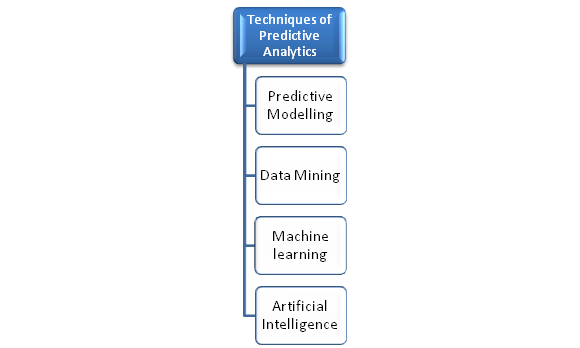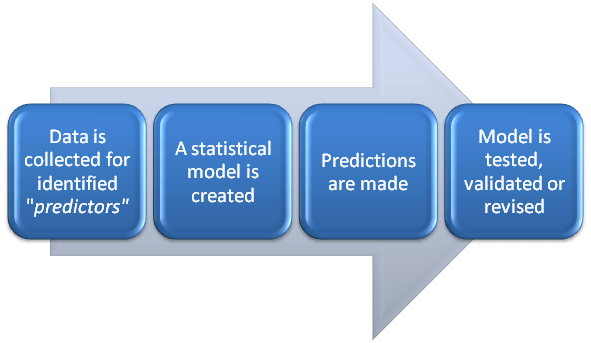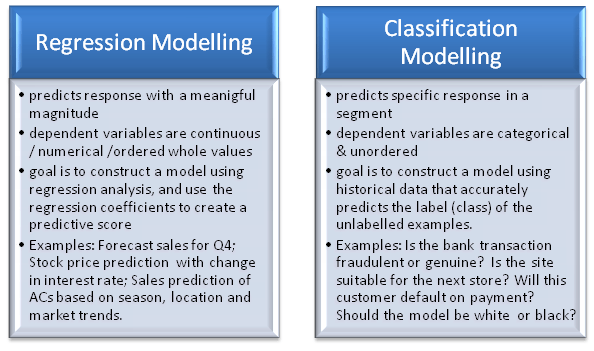# Modelling Techniques in Predictive Analytics

Analytics aims to understand the ‘why’ if you lose a customer. Predictive analytics moves beyond this to prevent you from losing a customer before it happens.

Predictive analytics supports insight, not hindsight. You discover meaningful trends and patterns in the data, and make predictions of unknown future outcomes. Using real world models that leverage mathematical and/or statistical techniques, you can predict possible events with a fair degree of accuracy.Models, in predictive analytics, represent meaningful relationships among variables, response or explanatory variables. Response variable refers to the quantity about which a query is made, while explanatory variable is the factor influencing the response variable. Explanatory variables are useful in predictive models – for observation, manipulation and control in relation to response. Patterns in historical and transactional data are studied for such variables to draw conclusions and make forecasts or identify opportunities.

Predictive Modeling

Predictive modeling is the creating, testing, validating and evaluating of a model to predict an outcome with a given input data.

A predictive model has predictors, i.e. variables likely to influence future outcomes. For instance, the gender, age group, location and purchase history of a customer adds up to the probability of a repeat transaction. These are ‘predictors‘.What does a Predictive model do?

• represents relationships in historical or/and real-time data
• looks for useful predictors or explanatory variables in the given data set
• fits models to subsets of data for evaluation of each predictor
• makes observations, forecasts, classifications, predictions about future events.
• assigns metrics for future actions

Approaches of Predictive Modelling

1. Traditional – driven by theories; uses classical or Bayesian methods of statistical inference; estimates parameters for linear predictors
2. Data-adaptive – driven by data; searches the data for subsets of useful predictors using machine learning methods; establishes non-linear relationships and interactions among variables
3. Model dependent – driven by specific model; uses the model/simulations to generate data, predictions or make recommendations; generated data is further compared with real data

Common predictive models are regression and classification.Look out for our next post on  “Common Statistical techniques used in Predictive modelling”.